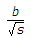# Civil Engineering - Theory of Structures

Exercise : Theory of Structures - Section 4
6.
The force in BC of the truss shown in the given figure, is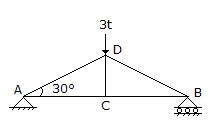3.0t compression
3.0t tension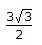t tensiont compression
None of these.
Explanation:
No answer description is available. Let's discuss.

7.
The equivalent length of a column of length L, having one end fixed and other end hinged, is
2L
L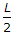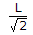Explanation:
No answer description is available. Let's discuss.

8.
At any point of a beam, the section modulus may be obtained by dividing the moment of inertia of the section by
depth of the section
depth of the neutral axis
maximum tensile stress at the section
maximum compressive stress at the section
none of these.
Explanation:
No answer description is available. Let's discuss.

9.
For calculating the permissible stress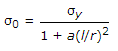is the emprical formula, known as
Straight line formula
Parabolic formula
Perry's formula
Rankine's formula.